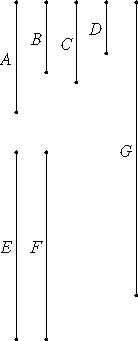# Proposition 19

If four numbers are proportional, then the number produced from the first and fourth equals the number produced from the second and third; and, if the number produced from the first and fourth equals that produced from the second and third, then the four numbers are proportional.

Let A, B, C, and D be four numbers in proportion, so that A is to B as C is to D, and let A multiplied by D make E, and let B multiplied by C make F.I say that E equals F.

Multiply A by C to make G. Since, then, A multiplied by C makes G, and multiplied by D makes E, therefore the number A multiplied by the two numbers C and D makes G and E. Therefore C is to D as G is to E. But C is to D as A is to B, therefore A is to B as G is to E.

VII.18

Again, since A multiplied by C makes G, but, further, B multiplied by C makes F, therefore the two numbers A and B multiplied by a certain number C make G and F. Therefore A is to B as G is to F.

(V.11)
(V.9)

But further A is to B as G is to E, therefore G is to E as G is to F. Therefore G has to each of the numbers E and F the same ratio. Therefore E equals F.

Again, let E equal F.

I say that A is to B as C is to D.

(V.7)

With the same construction, since E equals F, therefore G is to E as G is to F.

But G is to E as C is to D, and G is to F as A is to B, therefore A is to B as C is to D.

Therefore, if four numbers are proportional, then the number produced from the first and fourth equals the number produced from the second and third; and, if the number produced from the first and fourth equals that produced from the second and third, then the four numbers are proportional.

Q.E.D.

## Guide

Symbolically,

a : b = c : d if and only if ad = bc.

These algebraic expressions are meaningful when the variables are all numbers, but not when they are magnitudes in general. They can be interpreted, however, when they are lines, and proposition VI.16 is the analogue in that case.

Twice in this proof Euclid makes conclusions about proportions for numbers that he has neither stated nor proved. These places are indicated by (V.11), (V.9), and (V.7) in the margins, the analogous justifications for magnitudes. Some of the propositions in Book V for magnitudes are stated in proved in Book VII for numbers, in particular, V.16 and VII.13 correspond, and V.22 and VII.14 correspond. But many of the propositions in Book V have no analogue in Book VII, such as V.11, V.9, and V.7.

Now it could be that Euclid considered the missing statements as being obvious, as Heath claims, but being obvious is usually not a reason for Euclid to omit a proposition. Furthermore, other propositions in the next three books assume properties about proportions of numbers without having proofs of those propositions. One explanation is that the books on number theory, including this one, are older, and when the material in Book V was developed by Eudoxus, or when it was incorporated into the Elements by Euclid, more careful attention was made to fundamental propositions like V.7, V.9, and V.11.

This proposition is used frequently in Books VII and IX starting with VII.24.